# 前言

一句话概括一下ascon加密算法，它是NIST（美国国家标准与技术研究院）在凯撒加密竞赛中评选出最新的适用于IoT的一个加密算法家族。

既然他是一个算法家族，那么肯定不止一个算法，准确来说是参数不同，算法过程基本相同。请看基本介绍

更多关于ASCON的知识，请看这些网址

• Python版本的，由官方人员编写，Ascon-Python
• Java版本的，由官方人员编写，Ascon-Java
• C版本的，由官方人员编写，Ascon-C
• C++版本的，由我编写，Ascon-C++

# 基本介绍

## 参数推荐

先跳过这一部分，可以看下面的，之后过来查。官方推荐是ASCON-128 + ASCON-HASH组合更好一点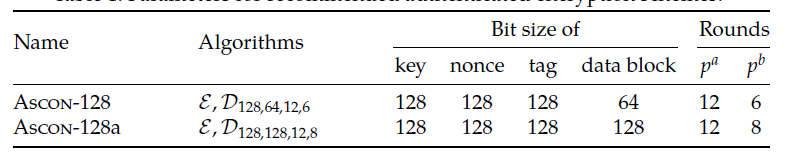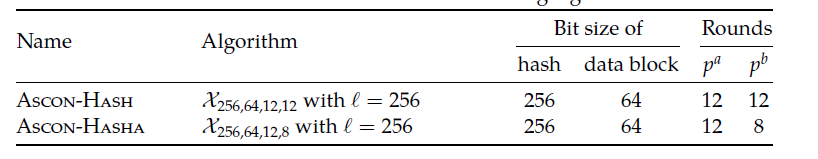## 符号介绍

这一步分也先跳过，看后面的，之后过来查

K 密钥，长度（提交给NIST的论文说是160bits以内，而谷歌学术-提交给凯撒协会的论文确实128bits以内，我没有确定好是哪一个）
N 随机数，128bits
T 标志，用于认证的，128bits
M,H 消息M，哈希值H

S 海绵结构的320bit状态S
Sr,Sc 状态S的rate和capacity部分，一个S是320bits，前面r比特是$S_r$，后面c比特是$S_c$$r+c=320$
$p,p^a,p^b$ 全排列过程，见$p^a(),p^b()$过程
$x\in \{0,1\}^k$ 比特流x，长度k（如果k是*的话，表示长度是变量）
$0^k$ 比特流，长度为k，值全0（如果k是*的话，表示长度是变量）
|x| 比特流x的长度，单位是bits
$\lfloor x\rfloor_k$ 将比特流x截断，只取前k位
$\lceil x\rceil^k$ 讲比特流x截断，只取后k位
x||y 比特流x与比特流y拼接起来
$x\oplus y$ 比特流x与比特流y异或
$x \mod y$ x除以y的余数
$\lceil x\rceil$ 向上取整
$p_C,p_S,p_L$ 上述$p$过程分出的三个子过程
$x_0,...,x_4$ 状态S五组64bits的数据，状态S是320bits，分为5组，每组64bits
$x_{0,i}\quad,...,\quad x_{4,i}$ 表示上面五组数据中的第i位，比如$x_{0,52}$表示$x_0$的第52哥数据（从0开始），其中$x_{.,0}$表示最右边的那位
$x\bigodot y$ 同或
$x >\!\!>\!\!> i$ 比特流x循环右移i位

## 加密/解密流程

• Initialization：初始化
• Processing Associated Data：处理关联数据A的过程
• Processing Plaintext/Ciphertext：处理明文（加密）/密文（解密）的过程
• Finalization：终止化

下面是流程图，看不懂也没关系，我也没看懂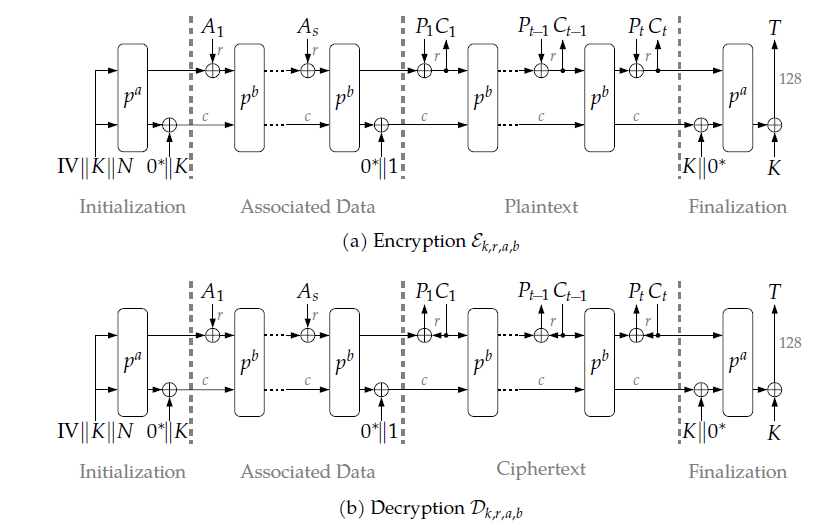下面两个公式无关紧要：

$\mathcal{\epsilon}_{a,b,k,r}(K,N,A,P)=(C,T)$

$\mathcal{D}_{a,b,k,r}(K,N,A,C,T)\in\{P,\perp\}$

# 过程详解

因为填充和全排列可能会在过程中遇到，所以想先讲讲这两个怎么实现吧，注意，下面所有的过程都是以ASCON-128ASCON-HASH作为讲解。

## 填充Padding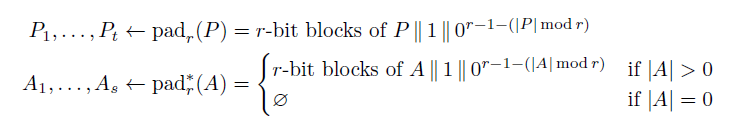以明文举个例子，需要对明文填充一个1$r-1-(|P| \mod r)$0，然后再将整个拼接好的串分为t组，每组rbits，关联数据A同理可得。密文是不需要填充的，因为填充的部分也不会用到。

## 全排列Permutation

$p$过程由$p_C,p_S,p_L$三个过程组合而成的。

• 下面是对状态S的两种分组过程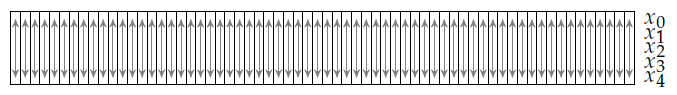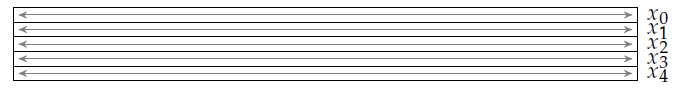• 分组：上述两图，一个是分成64组，一个是分成5组。
• 增加常量$p_C$：分成5组。只操作$x_2$，常量为$c_r$，使得$x_2=x_2\oplus c_r$，常量选择见下图（常量其实是有规律的，高四位是由F递减，低四位是由0递增）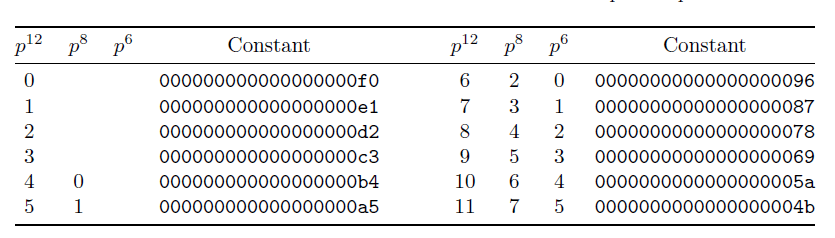• 增加常量$p_C$：可以简化成下列公式
• $x_2 = x_2 \oplus ( (15-i) << 4 ) | i$，其中$i=6\rightarrow 11$或者 $i=0\rightarrow 11$，这取决于执行次数，前者是执行6次，后者是执行12次。
• 替换$p_S$：原理介绍 （实现过程中一般不看这里，这里只是原理）
• 按照分成64组的分法，每5bits为一组，按照下表进行替换。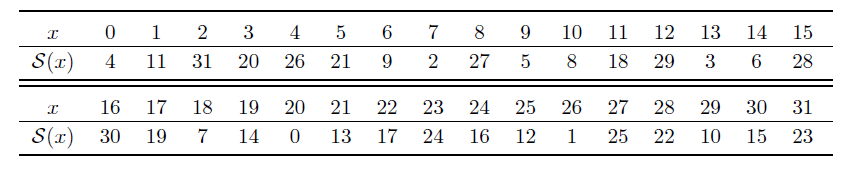• 替换$p_S$：简化替换$p_S$，以便数字电路也能执行，这里依然是分成5组的方法
• 使用下列数字电路进行替换，方便在FPGA上实现，同时给出了公式（公式中的$t_i$代表是临时变量）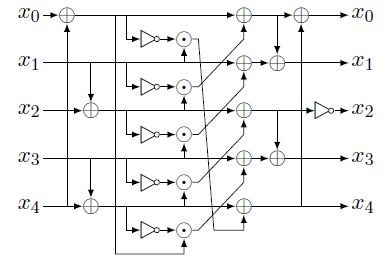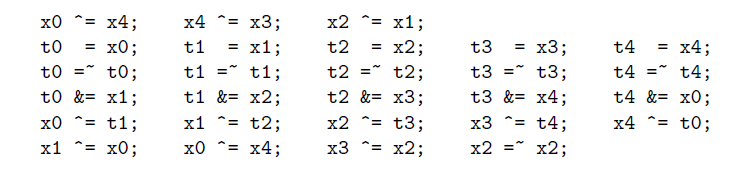• 线性扩散$p_L$：依然是分成5组。注意是循环右移，而不是单纯的右移

$x_0 = x_0 \oplus (x_0 >\!\!>\!\!> 19) \oplus (x_0 >\!\!>\!\!> 28) \\ x_1 = x_1 \oplus (x_1 >\!\!>\!\!> 61) \oplus (x_1 >\!\!>\!\!> 39) \\ x_2 = x_2 \oplus (x_2 >\!\!>\!\!> 1) \oplus (x_2 >\!\!>\!\!> 6) \\ x_3 = x_3 \oplus (x_3 >\!\!>\!\!> 10) \oplus (x_3 >\!\!>\!\!> 17) \\ x_4 = x_4 \oplus (x_4 >\!\!>\!\!> 7) \oplus (x_4 >\!\!>\!\!> 41)$

## 初始化Initialization

• 状态S的构成，状态S是320bits$S=Ⅳ||K||N$，注意这里是没有数据（明文、密文/关联数据）的
• 介绍上面的Ⅳ：如下图所示，我们还可以知道k,r,a,b分别是多少，其中k=128,r=64,a=12,b=6Ⅳ总共是占64bits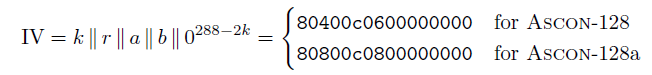• $S = p^a(S)\oplus(0^{320-k}||K)$，其中$p^a(S)$请参考上述过程，注意执行轮次有$p^a=12$

## 处理关联数据Processing Associated Data

• 填充数据中知道，关联数据A需要被分成s份，每一份是rbits
• $S=p^b((S_r\oplus A_i)||S_c),\quad 1 \leq i \leq s$，注意$p^b()=6$次，$S_r$代表$S$的前$r=64$bits$S_c$代表$S$的后$c=320-r=256$bits，其实$S$的长度没有变的
• $s$轮执行完之后，需要$S=S\oplus (0_{319}||1)$一下

## 处理密文/明文Processing Plaintext/Ciphertext

### 加密

• 填充数据中知道，明文P需要被分成t份，每一份是rbits。其中r=64
• 对于 $i=1\rightarrow (t\!-\!1)$
• $C_i = S_r \oplus P_i$
• $S = p^b (C_i \| S_c)$，其中 $S_c$表示状态S的后c=256比特数据
• 对于 $i=t$
• $C_t = S_r \oplus P_t$
• $S = C_i \| S_c$
• 对于最后一个密文块$C_t$需要特殊处理：截取前几位，舍弃后面的
• $C_t = \lfloor C_t \rfloor_l$，其中$l = |P|\ mod\ r$
• 这是因为明文是填充过的，如果不截取前几位，那么密文有可能比明文长，所以需要截取前 $|P|\ mod\ r$位数据

### 解密

• 密文不需要填充，但是仍然分成t组，除最后一组外每组r=64比特，最后一组是$l = |C| \ mod\ r$比特。
• 对于$i=1 \rightarrow (t\!-\!1)$
• $P_i = S_r \oplus C_i$
• $S = p^b(C_i||S_c)$：将状态S前r比特替换成$C_i$，然后执行$p^b$过程
• 对于$i=t$
• $P_t = \lfloor S_r \rfloor_l \oplus C_t$：这是因为$C_t$的长度只有$l$比特了，因此只需要用$S_r$的前$l$比特就行。
• $S=C_t||(\lceil S_r \rceil ^ {r-l} \oplus (1||0^{r-1-l}))||S_c$：这里其实就是把状态S前$l$比特替换成$C_t$，将第$l+1$比特与1异或。

## 终止化Finalization

$S = p^a(S\oplus (0^r || K || 0^{c-k}))$

$T = \lceil S\rceil ^k \oplus K$

• 加密过程中，返回标志T和密文
• 解密过程中，返回标志T和明文，但是只有当计算的表示T和收到的标志T匹配才有效

# 哈希过程

哈希过程另外开了一篇博客讲解，请移步至Ascon-Hash过程详解

# 综合讲解## 输入参数讲解

• 密钥K就不说了，就是你的密码
• 随机数N，这个也没啥好说的，用一个东西产生随机数即可
• 标志T，用来验证解密是否正确的，由加密方提供给解密方
• 明文/密文P/C，这个更不用说
• 关联数据A，这个是什么呢？相当于你的账号，然后密钥相当于你的密码

# 代码实现

本章节实现AsconV12，主要是为了讲解如何实现，使用的语言是C++。

## 定义数据类型

明文，密文和关联数据都涉及两个数据类型

• 一个数据类型是用户接口的，为ascon_data，外部进行传参
• 一个数据类型是内部实现，考虑到实现过程中需要将明文，密文和关联数据进行分组，分成 若干组，每组64bits，参考填充章节

状态S，则只有一个类型

• 状态S定义为ascon_state，它大小固定，为5行，每行都是一个64bits的数据

随机数与标志都是使用自定义的ascon_128，因为他们是固定的128位

• 在状态S中是占两行
#include <vector>
// 定义单个字节数据，C++实现并不是使用bit作为最小数据单位。
using ascon_8 = unsigned char;
// 定义64bits数据。
using ascon_64 = unsigned long long int;
// 定义明文，密文和关联数据原始类型。
using ascon_data = std::vector<ascon_8>;
// 算法运算过程中，定义状态S的数据类型。5行，每行64bits
using ascon_state = std::vector<ascon_64>;
// 算法运算过程中，定义明文，密文和关联数据类型。行数不知，每行64bits
// 定义 Permutations 的类型，分为a和b
using permutations_type = int;

// 实现一个128位的类，原型如下，可以选择把数据设置为私有类型
// 实现思路：使用两个64位数据组合而成
class ascon_128 {
public:
ascon_128();
ascon_128(ascon_64, ascon_64);
ascon_64 high, low;
void copyto64(ascon_64& a, ascon_64& b) const;   // 复制到两个64位的数据中去
void xorwith64(ascon_64& a, ascon_64& b) const;  // 异或到两个64位的数据中去
void showhex(std::ostream& out) const;   // 以显示十六进制显示
bool operator==(const ascon_128& tmp)const;   // 判断两个128位的数是否相等
};
// ascon_128 实现如下：
ascon_128::ascon_128():high(0), low(0) { } // 默认初始化为0
ascon_128::ascon_128(ascon_64 a, ascon_64 b) :high(a), low(b) {}

void ascon_128::copyto64(ascon_64& a, ascon_64& b) const {
a = high;
b = low;
}
void ascon_128::xorwith64(ascon_64& a, ascon_64& b) const {
a ^= high;
b ^= low;
}
void ascon_128::showhex(std::ostream& out) const {
out << std::hex << high << low;
}
bool ascon_128::operator==(const ascon_128& tmp)const {
return (tmp.high == this->high) && (tmp.low == this->low);
}


## 定义常量

// 定义常量数据
// 注意：下面的数据类型我都定义在一个类Asconv12里面
// 注意：因为为了我之后能够移植到C语言，所以并没有定义成员变量，并且都是定义的静态成员和函数
class Asconv12 {
public:
Asconv12();
static void encryption(
const ascon_data& plaintext,
const ascon_data& associatedData,
ascon_data& ciphertext,
const ascon_128& keys,
const ascon_128& nonce,
ascon_128& T
);
static bool decryption(
const ascon_data& ciphertext,
const ascon_data& asconciatedData,
ascon_data& plaintext,
const ascon_128& keys,
const ascon_128& nonce,
ascon_128& T
);
private:
static void permutations(permutations_type t, ascon_state &S);

// 循环右移
static ascon_64 ROTR(ascon_64 d, int n);

// 过程
static void Initalization(ascon_state& S, const ascon_128& keys, const ascon_128& nonce);
static void ProcessingAssociatedData(ascon_state& S, ascon_padding& A,
const ascon_data& associatedData);
static void ProcessingPlaintext(ascon_state& S, ascon_padding& P,
ascon_data& ciphertext);
static void ProcessingCiphertext(ascon_state& S, ascon_padding& C,
ascon_data& plaintext);
static bool Finalization(ascon_state& S, const ascon_128& keys,
ascon_128& T, const ascon_128& Ttmp = { 0, 0 });

// 将内部的数据类型转成外部接口的数据类型输出
static void transform(const ascon_padding& in, ascon_data& out, size_t l);

// 定义常量数据
static const permutations_type a = 12;  // permutatuions_type见上个小节
static const permutations_type b = 6;

static const int k = 128;  // 这里的单位都是：bits，这个后面没怎么用到
static const int r = 64;
static const int c = 256;  // 这个后面没有怎么用到
};


## 实现填充过程

// 明确数据类型，是从ascon_data转成ascon_padding，也就是外界接口的数据类型转成内部实现的数据类型
// need是表示是否需要填充，明文，关联数据都是需要填充的，指填充 100000...0
// 设置为false就是不填充，密文是不填充
if (data.size() == 0) return;

// 讲一下one_zero_Bytes，填充过程中后面需要填充 1000...000 的字节数看，上述公式就行
size_t one_zero_Bytes = (Asconv12::r - ((data.size() * 8) % Asconv12::r)) / 8;
one_zero_Bytes = one_zero_Bytes == 0 ? 1 : one_zero_Bytes;  // 一定要填充，而不能不填充

// 因为它是每行64bits=8Bytes，所以需要除以每行大小
size_t size_of_out = need ? (data.size() + one_zero_Bytes) / 8 : (data.size() / 8 + (data.size() % 8 > 0));

if (!out.empty()) out.clear();
out.resize(size_of_out);
std::fill(out.begin(), out.end(), 0);   // 将所有填充为0，作为初始化

// 填充时没有使用单独的一片空间将填充数据加进去，所以在这个过程进行添加
// ascon_data mod 8并不是为0的，所以最后一行特殊处理
for (size_t i = 0; i < size_of_out - 1; i++) {
for (int j = 0; j < 8; j++) {
out[i] <<= 8;
out[i] |= (ascon_64)data[i * 8 + j];
}
}

// 最后一行特殊处理，
for (int i = 0; i < 8; i++) {
size_t index = (size_of_out - 1) * 8 + i;
out[size_of_out-1] <<= 8;
if (index < data.size()) {
out[size_of_out - 1] |= (ascon_64)data[index];
}
else if (index == data.size()) {
if(need)
out[size_of_out - 1] |= (ascon_64)0x80;
}
}
}


## 实现Permutations

// 在此之前，先实现一下循环右移，其实很简单的，自己推一下就明白了
ascon_64 Asconv12::ROTR(ascon_64 d, int n) {
return ((d << (64 - n)) | (d >> n));
}

// permutations，t表示a过程还是b过程，其实就是次数不同
// 状态S时运行过程中的
void Asconv12::permutations(permutations_type t, ascon_state& S) {
ascon_64 x0 = S, x1 = S, x2 = S, x3 = S, x4 = S, t0, t1, t2, t3, t4;
for (ascon_64 i = 12 - t; i < 12; i++) {
// 常量添加，注意看上述过程讲解时，常量是有规律的，这个公式也是看的官方的
x2 ^= (((ascon_64)(0xf) - i) << 4) | i;

// 替换层，直接根据公式来的
x0 ^= x4;    x4 ^= x3;    x2 ^= x1;
t0  = x0;    t1  = x1;    t2  = x2;    t3  = x3;    t4  = x4;
t0 = ~t0;    t1 = ~t1;    t2 = ~t2;    t3 = ~t3;    t4 = ~t4;
t0 &= x1;    t1 &= x2;    t2 &= x3;    t3 &= x4;    t4 &= x0;
x0 ^= t1;    x1 ^= t2;    x2 ^= t3;    x3 ^= t4;    x4 ^= t0;
x1 ^= x0;    x0 ^= x4;    x3 ^= x2;    x2 = ~x2;

// 线性扩散层，直接根据公式来就行
x0 ^= Asconv12::ROTR(x0, 19) ^ Asconv12::ROTR(x0, 28);
x1 ^= Asconv12::ROTR(x1, 61) ^ Asconv12::ROTR(x1, 39);
x2 ^= Asconv12::ROTR(x2, 1) ^ Asconv12::ROTR(x2, 6);
x3 ^= Asconv12::ROTR(x3, 10) ^ Asconv12::ROTR(x3, 17);
x4 ^= Asconv12::ROTR(x4, 7) ^ Asconv12::ROTR(x4, 41);
}
// 更新状态S
S = x0; S = x1; S = x2; S = x3; S = x4;
}


## 数据类型转换

考虑到内部实现中所用的数据类型是类二维的，外部接口都是一维的，所以需要转换

// ascon_padding 转 ascon_data，内部的转外部
void Asconv12::transform(const ascon_padding& in, ascon_data& out, size_t l) {
if (!out.empty()) out.clear();
// output's Bytes
size_t out_size = ((in.size() - 1) * 64 + l) / 8;
out.resize(out_size);

// 最后一行单独处理，因为最后一行其实只有lbits数据，不是1是l
for (int i = 0; i < in.size() - 1; i++) {
for (int j = 0; j < 8; j++) {
out[i * 8 + j] = (ascon_8)(in[i] >> ((7 - j) * 8));
}
}

for (int i = 0; i < l / 8; i++) { // 最后一行数据特殊处理，只有 l/8个字节的数据
out[8 * (in.size() - 1) + i] = (ascon_8)(in[in.size() - 1] >> ((7 - i) * 8));
}
}


## Initialization

// 不要纠结单词打错了
// 初始化
void Asconv12::Initalization(ascon_state& S, const ascon_128& keys, const ascon_128& nonce) {
if (!S.empty())S.clear();
S.resize(5);
S = 0x80400c0600000000; // Ⅳ：k || r || a || b || 0^{288-2k}，就固定使用就行
keys.copyto64(S, S);
nonce.copyto64(S, S);

Asconv12::permutations(Asconv12::a, S); // p^a操作

keys.xorwith64(S, S); //
}


## Processing Associated Data

// 处理关联数据
void Asconv12::ProcessingAssociatedData(
ascon_state& S,   // 状态S
const ascon_data& associatedData // 外部传入的关联数据
){
if (!A.empty()) {
for (int i = 0; i < A.size(); i++) {
S ^= A[i];
Asconv12::permutations(Asconv12::b, S);
}
S ^= (ascon_64)0x1;
}
}


## Processing Plaintext

// 处理明文
void Asconv12::ProcessingPlaintext(
ascon_state& S, // 状态S
const ascon_data& plaintext, // 外部输入的明文
ascon_data& ciphertext // 输出的密文
) {
if (!C.empty()) C.clear();
C.resize(P.size());
// 计算l
size_t l = plaintext.size() * 8 % Asconv12::r;
for (int i = 0; i < P.size(); i++) {
C[i] = S ^ P[i];
S = C[i];
if (i < P.size() - 1) {  // 处理前 t-1 个数据
Asconv12::permutations(Asconv12::b, S);
}
else { // 最后一个数据特殊处理
C[i] &= ((0xFFFFFFFFFFFFFFFF >> (64 - l)) << (64 - l));
}
}

Asconv12::transform(C, ciphertext, l);
}


## Process Ciphertext

// 处理密文
void Asconv12::ProcessingCiphertext(
ascon_state& S,  // 状态S
const ascon_data& ciphertext, // 外部数据的密文数据
ascon_data& plaintext // 输出到外部的明文数据
) {
if (!P.empty()) P.clear();
P.resize(C.size());
for (int i = 0; i < C.size()-1; i++) {
P[i] = S ^ C[i];
S = C[i];
Asconv12::permutations(Asconv12::b, S);
}
int index = C.size() - 1;
size_t l = ciphertext.size() * 8 % Asconv12::r;

// 这一段看公式自己写会更好
// 本质也就是操作S前r个数据也即是S
P[index] = ((S >> (64 - l)) << (64 - l)) ^ C[index];
S = C[index] // Ct是前面l个数据加r-l个0，
|  // 取或就相当于拼接
(((S << l) >> l) ^ ((ascon_64)1 << (63 - l)));

// 感觉不太好解释，但是反正实现那个公式肯定不止这一种方法的

Asconv12::transform(P, plaintext, l);
}


## Finalization

// 终止化
bool Asconv12::Finalization(
ascon_state& S, // 状态S
const ascon_128& keys, // 密钥
ascon_128& T,  // 计算出来的标志
const ascon_128& Ttmp // 加密过程这个参数用不到，或者说不管他，解密过程表示接收到的标志
) {
keys.xorwith64(S, S);
Asconv12::permutations(Asconv12::a, S);
T.high = S ^ keys.high;
T.low = S ^ keys.low;
return T==Ttmp; // 解密的时候需要判断一下标志是否相等
}


## 加密和解密

调用上述过程就行

void Asconv12::encryption(const ascon_data& plaintext, const ascon_data& associatedData, ascon_data& ciphertext, const ascon_128& keys, const ascon_128& nonce, ascon_128& T) {
ascon_state S;
// 初始化
Asconv12::Initalization(S, keys, nonce);
// 处理关联数据
Asconv12::ProcessingAssociatedData(S, A, associatedData);
// 处理明文
Asconv12::ProcessingPlaintext(S, P, C, plaintext, ciphertext);
// 终止化
Asconv12::Finalization(S, keys, T);
}

bool Asconv12::decryption(const ascon_data& ciphertext, const ascon_data& assonciatedData, ascon_data& plaintext, const ascon_128& keys, const ascon_128& nonce, ascon_128& T) {

ascon_state S;

// 初始化
Asconv12::Initalization(S, keys, nonce);
// 处理关联数据
Asconv12::ProcessingAssociatedData(S, A, assonciatedData);
// 处理密文
Asconv12::ProcessingCiphertext(S, C, P, ciphertext, plaintext);
// 终止化
ascon_128 Tout;
bool flag = Asconv12::Finalization(S, keys, Tout, T);

return flag;
}


# 结束语

这个算法是一个比较新颖的算法，至今国内还没有很多的资料。考虑到毕设可能用到这个，所以特地写了一份参考教程。要是有问题就在评论区问吧，我也是才学，可以一起 交流交流。